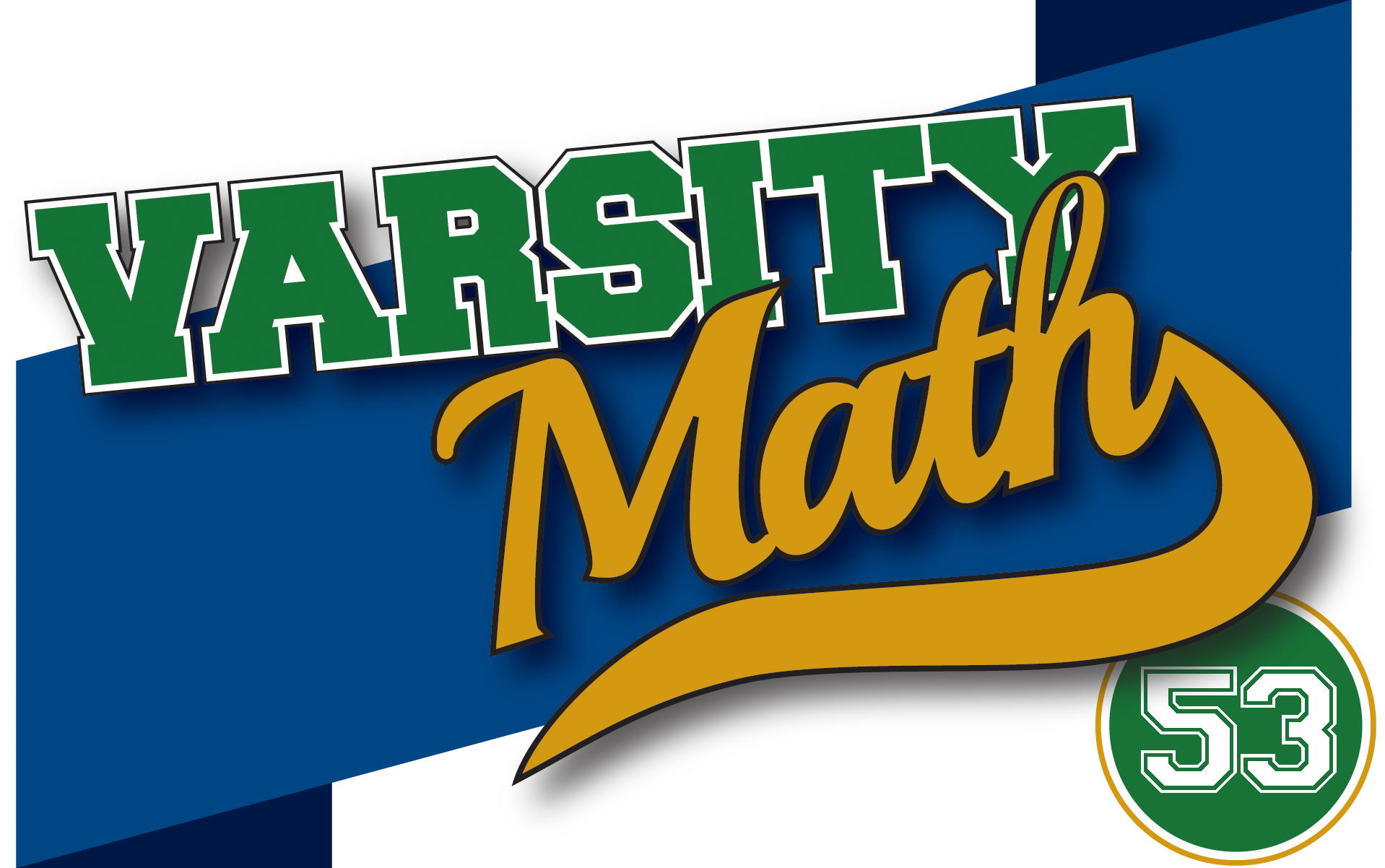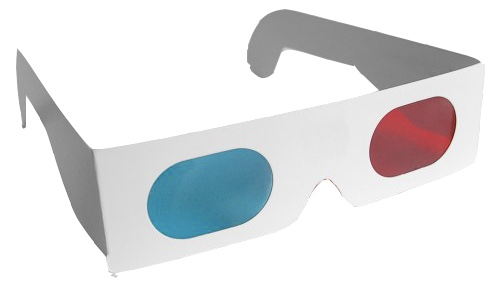## ________________

When Coach Newton announces that the first practice of the year will be on fractions, the students complain, “Awww! We’re way beyond that!” “Don’t worry,” consoles the coach, “these fractions come from the third dimension.”

## ________________Yoshi is idly drawing diagonals on the faces of a cube, and suddenly realizes that there are two configurations in which six corner-to-corner diagonals, one on each face of the cube, form the six edges of a triangular pyramid.

What fraction of the volume of the cube is either pyramid?

### Hexintersection

T is a regular tetrahedron with edge length equal to 3 units. Sphere S is centered at the midpoint of one edge of T and has radius 3/2 units. S intersects the edges of T at six points which can be viewed as the vertices of a solid H with three quadrilateral faces and two triangular faces.

What fraction of the volume of T is the volume of H?

## Solutions to week 52

Mean Triangle. If you draw a diagram of the situation as shown, you can see that any right triangle together with a copy rotated 180° about the midpoint of the hypotenuse forms a rectangle, and so the length of the median to the hypotenuse is half the length of the hypotenuse (since it is half the diagonal of the rectangle). In this problem, the length of that median is also the geometric mean of the legs, so if a is the length of the shorter leg, b the longer leg, and c = 10 the hypotenuse, then √ab = c/2 = 5. We also have the Pythagorean theorem: a² + b² = c² = 100. If we add twice the square of the first equation to the second, we get that (a + b)² = a² + b² + 2ab = 150, so a + b = √150 = 5√6. On the other hand, since b is longer than a, ba is positive, so (ba)² = a² + b² − 2ab = 50, and ba = √50 = 5√2. Subtracting the last equation from the prior one and dividing by two gives us that the desired length of the shorter leg is a = 5(√6 − √2)/2.

As Easy as 4132. Suppose we let p be the product of all four of the numbers in a sumo sequence. Then the defining property tells us that for every number x in the sequence, x + p/x = 2 (since the product of the other three numbers is just p/x). Solving this equation for x in terms of p, we see that x² – 2x + p = 0, or x = 1 ± √(1-p).

Now if p were equal to 1, then there would only be one solution to this equation, namely x = 1, and we get the valid sumo sequence (1,1,1,1). If p is anything else, then at most two different numbers can appear in the sumo sequence, namely the two roots of the above equation. If all of the numbers in the sequence happened to be the same, then we would in addition have that x4 = p. None of the elements in a sumo sequence can be zero, since then all of the others would have to be two, which does not work (because the product of three twos plus zero is eight, not two), so we can substitute x4 back into the quadratic equation and divide by x to get x³ + x² – 2 = 0. Further, since we have already found the case of all ones, we can also assume x is not one, and divide by x-1 to get x² + 2x + 2 = 0. This latter quadratic has no real roots (its discriminant b² – 4ac is negative), so we conclude that there are no more sumo sequences in which all of the terms are the same (but there would be more complex sumo sequences!).

Thus, if there is any other sumo sequence, it has product p ≠ 1; and it has one, two, or three terms equal to 1 – √(1-p) and the rest equal to 1 + √(1-p). If there were two of each, then the product of all of them would be p² (just multiply them all out, using the difference of two squares); but it is also just p. so that would mean that p² = p. This only has solutions p = 1 and p = 0, both of which we’ve already dealt with, so there are no new solutions with two of each possibility.

So we are left only with the possibility that we have one copy of one of the two numbers and three copies of the other. In this case, the product of one of each kind of number is (1 + √(1-p))(1 – √(1-p)) = p. Therefore, the product of the other two must be 1 (in order for the overall product to be p). But the other two are the same, so they must both be 1 or both be -1. Therefore, overall in the sequence there must be three ones or three minus ones. In the former case, the remaining number must be one, and we already have that solution. But in the latter case, if we have three minus ones, the other number must be 3, and that actually works, because not only is 3 + (-1)³ = 2, but also -1 + 3(-1)² = 2.

No other possibilities remain. Since there are four different orders in which one 3 and three -1s could appear in a sequence, we conclude there are exactly five sumo sequences.

Coach Newton chimes in:
Both of these problems share the common characteristic that in order to solve them, you have to introduce something that is not explicitly mentioned in the statement of the problem: the rectangle of which the triangle is half in Mean Triangle, and the product of all four numbers in the other problem. Finding that valuable stepping stone toward a solution is one of the keys to successful problem solving. The search for a good stepping stone can be frustrating, but finding it is one of the true joys of mathematics.

Oh, and if you happen to be wondering where the name of the second problem came from, it’s just that there are 4 numbers where 1 of them plus the product of the other 3 equals 2. How nice that the answer is the next whole number, 5.

## Recent Weeks

Links to all of the puzzles and solutions are on the Complete Varsity Math page.

Come back next week for answers and more puzzles.

[asciimathsf]